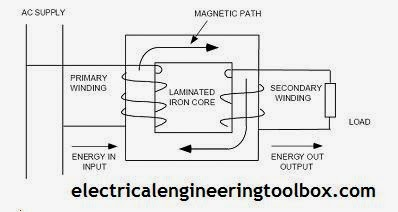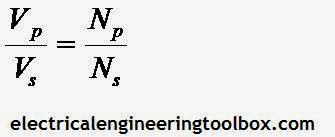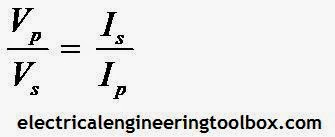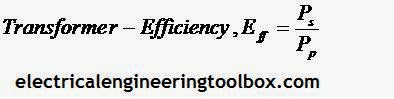### How a Voltage Transformer Works

Custom Search
Transformer function is based on the principle that electrical energy is transferred efficiently by magnetic induction from one circuit to another. Basically, a transformer consists of two or more windings placed on the same magnetic path. The winding being fed electrical energy is called the primary winding while the winding where the load is connected is called the secondary winding. A typical two winding transformer action is shown below:Transformer Action
When the primary winding of a transformer is energized from an alternating current (AC) source, an alternating magnetic field is established in the transformer core. Alternating magnetic lines of force, called “flux,” circulate through the core. With a second (secondary) winding around the same core, a voltage is induced by the alternating flux lines. A load, connected to the terminals of the secondary winding, results in current flow.

Parts of A Transformer
A transformer is made up of two basic non-moving parts:
(a) A laminated iron core
(b) Windings (primary & secondary)

Laminated Iron core
The iron core of a transformer is made up of sheets of rolled iron. This iron is treated so that it has a high magnetic conducting quality (high permeability) throughout the length of the core. Permeability is the term used to express the case with which a material will conduct magnetic lines of force.
The iron also has a high ohmic resistance across the plates (through the thickness of the core). It is necessary to laminate the iron sheets to reduce heating of the core. There are two common types of transformer cores:
(a) Core type
(b) Shell typeCore and Shell type transformers
In the core-type (core form) transformer, the windings surround the core. In a shell-type (shell form) transformer, the steel magnetic circuit (core) forms a shell surrounding the windings. In a core form, the windings are on the outside; in a shell form, the windings are on the inside.

Windings
A transformer has two windings; the primary winding and the secondary winding.
The primary winding is the coil which receives the energy.  It is formed, wound and fitted over the iron core. The secondary winding is the coil, which discharges the energy at a transformed or changed voltage.

Types of Transformers
Transformers are classified according to different criteria. However here is a list of the more common generic types of transformers:
(a) Single phase transformers
(b) Three phase transformers
(c) Potential or voltage transformers
(d) Autotransformers
(e) Current transformers
(f) Power transformers

Voltage Ratio of a Transformer
The voltage on the windings of a transformer is directly proportional to the number of turns on the coils of the windings. This relationship is expressed by the formula:Voltage ratio of a transformer
Where:
Vp = voltage on primary coils, V
Vs = voltage on secondary coils, V
Np = number of turns on primary coils
Ns = number of turns on secondary coils

The ratio Vp / Vs is called the voltage ratio (VR). The ratio Np / Ns is called the turns ratio (TR).

A voltage ratio of 1:4 (read as 1 to 4) means that for each volt on the transformer primary, there is 4V on the secondary. When the secondary voltage is greater than the primary voltage, the transformer is called a step-up transformer.

A voltage ratio of 4:1 means that for each 4V on the primary, there is only 1V on the secondary. When the secondary voltage is less than the primary voltage, the transformer is called a step-down transformer.

Current Ratio
The current in the coils of a transformer is inversely proportional to the voltage in the coils. This relationship is expressed by the equation:current ratio of a transformer

Where:
Ip = current in primary coil, A
Is = current in secondary coil, A

In the equation above we may substitute Np / Ns for Vp / Vs so we have:

Transformer Efficiency
The efficiency of a transformer is equal to the ratio of the power output of the secondary winding to the power input to the primary winding.
An ideal transformer is 100 percent efficient because it delivers all the energy it receives.
However, because of core and copper losses, the efficiency of even the best practical transformer is less than 100 percent. Expressed as an equation:Transformer Efficiency
Where:
Eff = efficiency
Ps  = power output from secondary = Input power –core losses – Copper losses
Pp  = power input to primary

Efficiencies of well-designed transformers are very high, averaging over 98 percent (%) for power transformers. The only losses, in a transformer, are due to core losses which go to maintaining the alternating magnetic field, resistance losses in the coils, and power used for cooling for large transformers requiring cooling.

The main reason for high efficiencies of transformers, compared to other equipment, is the absence of moving parts. Transformers are called static AC machines.